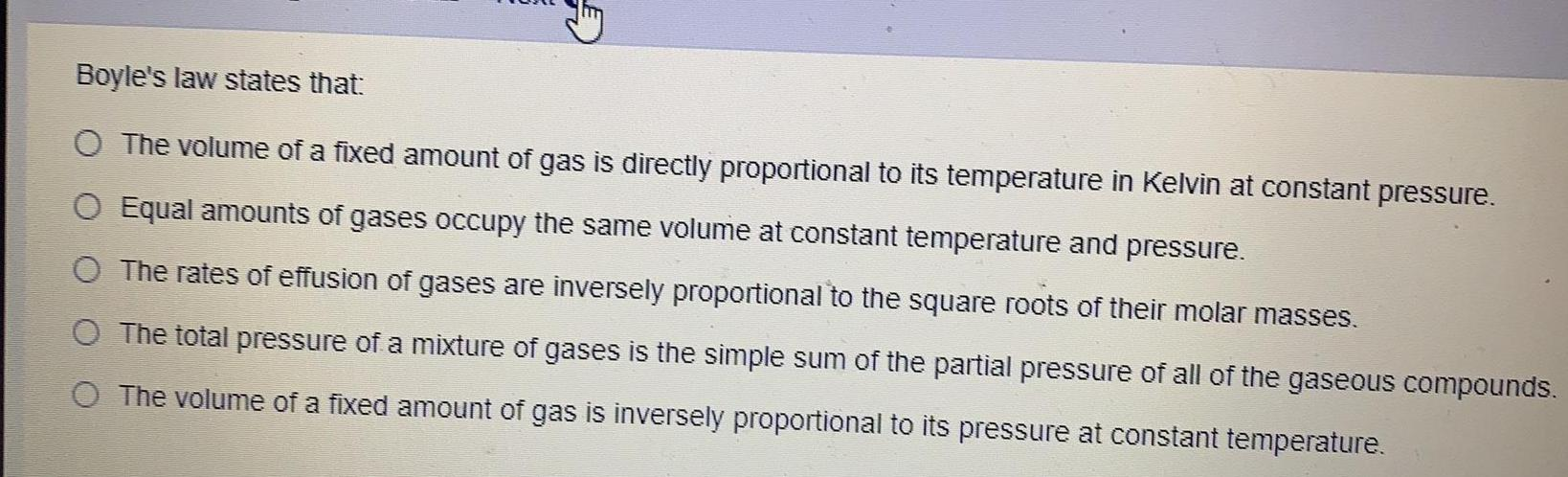Question:

# Boyle's law states that: The volume of a fixed amount of gas

Last updated: 7/29/2022Boyle's law states that: The volume of a fixed amount of gas is directly proportional to its temperature in Kelvin at constant pressure. Equal amounts of gases occupy the same volume at constant temperature and pressure. The rates of effusion of gases are inversely proportional to the square roots of their molar masses. The total pressure of a mixture of gases is the simple sum of the partial pressure of all of the gaseous compounds. The volume of a fixed amount of gas is inversely proportional to its pressure at constant temperature.